## Extract filename from a path in Excel

This tutorial explains how to apply formulas to extract filename with or without extension from a file path in a specific cell in Excel.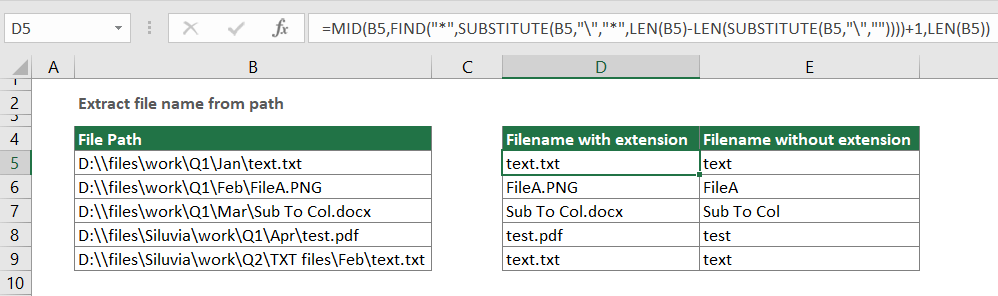Extract filename with extension from a path
Extract filename without extension from a path

#### Extract filename with extension from a path

As the below screenshot shown, there is a list containing different file paths, and you want to extract only the filenames with extensions from them, the following formula will help you solve the problem.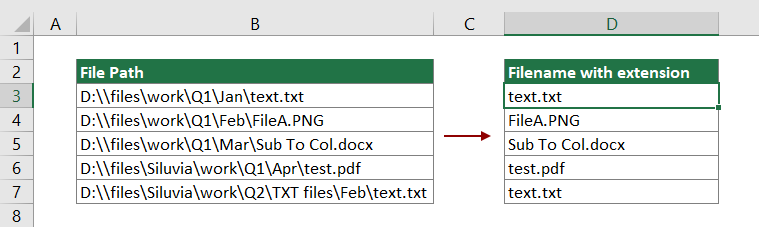Generic formula

=MID(A1,FIND("*",SUBSTITUTE(A1,"\","*",LEN(A1)-LEN(SUBSTITUTE(A1,"\",""))))+1,LEN(A1))

Arguments

A1: Represents the cell containing the file path you will extract the filename from it.

How to use this formula?

1. Select a blank cell to output the filename. In this case, I select the cell D3.

2. Enter the below formula into it and press the Enter key. Select the result cell, and then drag its Fill Handle all the way down to apply it to other cells.

=MID(B3,FIND("*",SUBSTITUTE(B3,"\","*",LEN(B3)-LEN(SUBSTITUTE(B3,"\",""))))+1,LEN(B3))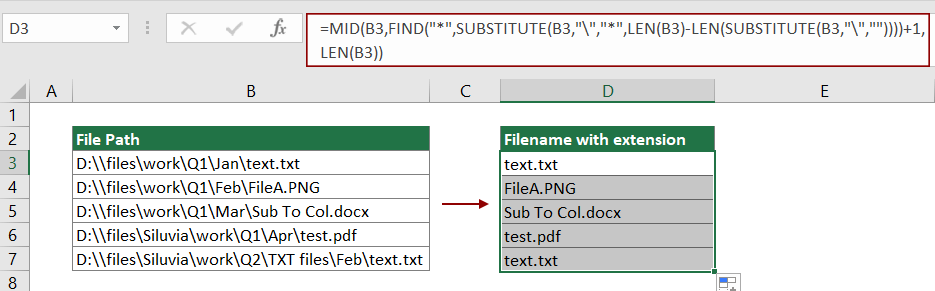Now all filenames with extensions in specific file paths are extracted as the above screenshot shown.

Notes:

• In this formula, B3 is the cell containing the file path. Please change it as you need.
• If the filename is no more than 99 characters long, the below shorter formula can also be used to solve the problem. See the below screenshot shown.
=TRIM(RIGHT(SUBSTITUTE(A2,"\",REPT(" ",100)),99))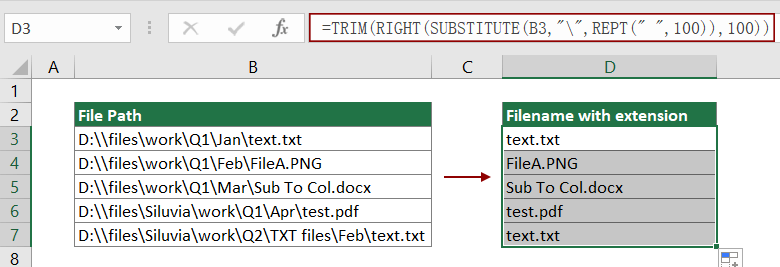How this formula works?

=MID(B3,FIND("*",SUBSTITUTE(B3,"\","*",LEN(B3)-LEN(SUBSTITUTE(B3,"\",""))))+1,LEN(B3))

1. FIND("*",SUBSTITUTE(B3,"\","*",LEN(B3)-LEN(SUBSTITUTE(B3,"\",""))))

• LEN(B3): The LEN function calculates the total length in "D:\\files\work\Q1\Jan\text.txt" and returns the result as 30;
• LEN(SUBSTITUTE(B3,"\","")): The SUBSTITUTE function replaces the character "\" with nothing. The result is "D:filesworkQ1Jantext.txt". And then the LEN function calculates the total length of "D:filesworkQ1Jantext.txt" and get the result 24;
• SUBSTITUTE(B3,"\","*",30-24): The SUBSTITUTE function replace the third character (30-24=6) "\" in "D:\\files\work\Q1\Jan\text.txt" with a single character "*". Here the result is "D:\\files\work\Q1\Jan*text.txt";
• FIND("*","D:\\files\work\Q1\Jan*text.txt"): The FIND function locates the position of character "*" in "D:\\files\work\Q1\Jan*text.txt" and finally returns 22. Here means that the character "*" is in the 22th position in "D:\\files\work\Q1\Jan*text.txt".

2. =MID(B3,22+1,LEN(B3))

• As the above explanation shown, LEN(B3) returns the result as 30, here the MID function can be shown as =MID("D:\\files\work\Q1\Jan\text.txt",23,30). It means that the MID function extracts 30 characters from the string "D:\\files\work\Q1\Jan\text.txt", starting at the 23rd character. Here the result is text.txt.

#### Extract filename without extension from a path

Another circumstance, you may just need to extract the filename without extension from a path as the below screenshot shown. This section will provide a long formula to help you get it down.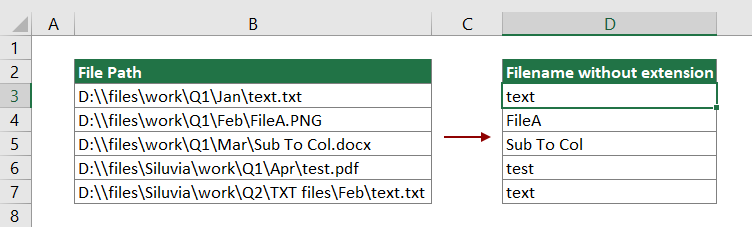Generic formula

=IFERROR(MID(A1,FIND(CHAR(1),SUBSTITUTE(A1,"\",CHAR(1),LEN(A1)-LEN(SUBSTITUTE(A1,"\",""))))+1,FIND(CHAR(1),SUBSTITUTE(A1,".",CHAR(1),LEN(A1)-LEN(SUBSTITUTE(A1,".",""))))-FIND(CHAR(1),SUBSTITUTE(A1,"\",CHAR(1),LEN(A1)-LEN(SUBSTITUTE(A1,"\",""))))-1),"")

Arguments

A1: Represents the cell containing the file path you will extract the filename from it.

CHAR(1): The CHAR(1) here can be replaced with any CHAR number as you need. Or it can be replaced with a specific symbol enclosed in quotation marks such as "*".

How to use this formula?

1. Select a blank cell to output the filename without the extension.

2. Enter the below formula into it and press the Enter key. Select the result cell, and then drag its Fill Handle all the way down to apply it to other cells.

=IFERROR(MID(B4,FIND(CHAR(1),SUBSTITUTE(B4,"\",CHAR(1),LEN(B4)-LEN(SUBSTITUTE(B4,"\",""))))+1,FIND(CHAR(1),SUBSTITUTE(B4,".",CHAR(1),LEN(B4)-LEN(SUBSTITUTE(B4,".",""))))-FIND(CHAR(1),SUBSTITUTE(B4,"\",CHAR(1),LEN(B4)-LEN(SUBSTITUTE(B4,"\",""))))-1),"")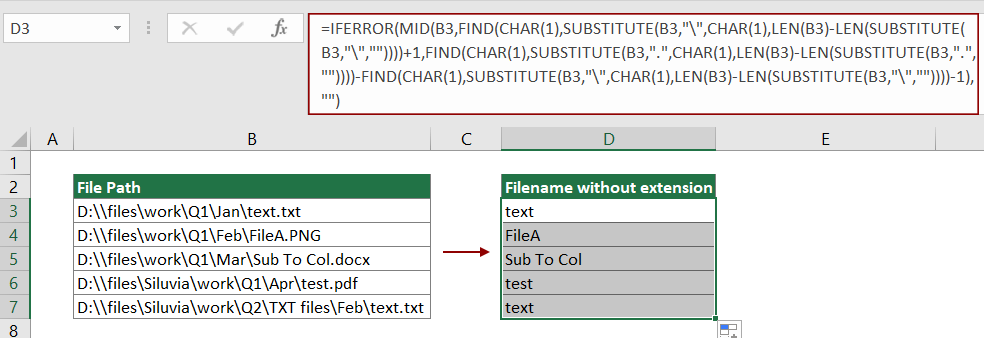Note: In this formula, B3 is the cell containing the file path. Please change it as you need.

#### Related functions

Excel MID function
The Excel MID function is used to find and return a specific number of characters from the middle of given text string.

Excel FIND function
The Excel FIND function is used to find a string within another string, and returns the starting position of the string inside another one.

Excel SUBSTITUTE function
The Excel SUBSTITUTE function replaces text or characters within a text string with another text or characters.

Excel LEN function
The Excel LEN function returns the number of characters in a text string.

Excel IFERROR function
The IFERROR function is used to return a custom result when a formula evaluates an error, and return a normal result when no error is occurred.

#### Related formulas

Extract Multiple Lines From A Cell
If you have a list of text strings which are separated by line breaks (that occurs by pressing Alt + Enter keys when entering the text), and want to extract these lines of text into multiple cells, the formula in this tutorial will do you a favor.

Extract Last Line Of Text From A Multi-Line Cell
To extract the last line of text from a text string which is separated by line breaks, a formula in this tutorial can help you deal with this task in Excel.

Extract Nth Word From Text String In Excel
This article explains how to use a formula to extract the specific nth word from a text string in a cell in Excel.

Extract Last Two Words From A Cell In Excel
This tutorial explains how to use formula to extract the last two words from a cell in Excel.

Extract Word Beginning With A Specific Character In Excel
This tutorial provides a formula with detail steps to help you extract the word which begins with a specific character from a text string in a cell in Excel.

Extract All Words But First Or Last
In this tutorial, you will learn how to use formulas to extract all words from a cell except the first or last one in Excel.

### The Best Office Productivity Tools

#### Kutools for Excel - Helps You To Stand Out From Crowd

 Popular Features: Find, Highlight or Identify Duplicates  |  Delete Blank Rows  |  Combine Columns or Cells without Losing Data  |  Round without Formula ... Super VLookup: Multiple Criteria  |  Multiple Value  |  Across Multi-Sheets  |  Fuzzy Lookup... Adv. Drop-down List: Easy Drop Down List  |  Dependent Drop Down List  |  Multi-select Drop Down List... Column Manager: Add a Specific Number of Columns  |  Move Columns  |  Toggle Visibility Status of Hidden Columns  |  Compare Columns to Select Same & Different Cells ... Featured Features: Grid Focus  |  Design View  |  Big Formula Bar  |  Workbook & Sheet Manager | Resource Library (Auto Text)  |  Date Picker  |  Combine Worksheets  |  Encrypt/Decrypt Cells  |  Send Emails by List  |  Super Filter  |  Special Filter (filter bold/italic/strikethrough...) ... Top 15 Toolsets:  12 Text Tools (Add Text, Remove Characters ...)  |  50+ Chart Types (Gantt Chart ...)  |  40+ Practical Formulas (Calculate age based on birthday ...)  |  19 Insertion Tools (Insert QR Code, Insert Picture from Path ...)  |  12 Conversion Tools (Numbers to Words, Currency Conversion ...)  |  7 Merge & Split Tools (Advanced Combine Rows, Split Excel Cells ...)  |  ... and more

Kutools for Excel Boasts Over 300 Features, Ensuring That What You Need is Just A Click Away...#### Office Tab - Enable Tabbed Reading and Editing in Microsoft Office (include Excel)

• One second to switch between dozens of open documents!
• Reduce hundreds of mouse clicks for you every day, say goodbye to mouse hand.
• Increases your productivity by 50% when viewing and editing multiple documents.
• Brings Efficient Tabs to Office (include Excel), Just Like Chrome, Edge and Firefox.